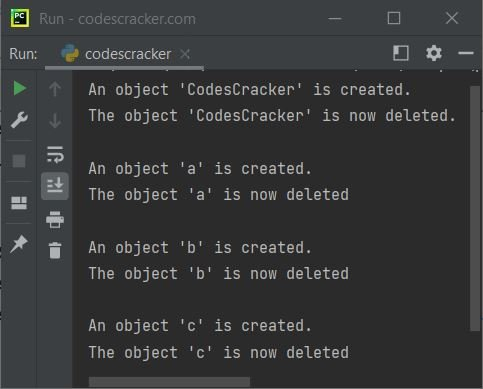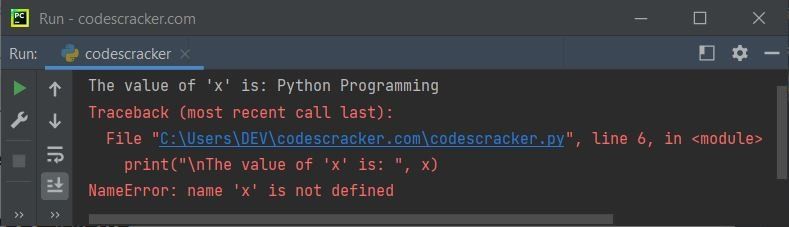# Python del Keyword

The del keyword in Python is used when we need to delete an object. For example:

```class CodesCracker:
Name = "William"
Age = "20"

print("An object 'CodesCracker' is created.")
del CodesCracker
print("The object 'CodesCracker' is now deleted.")

a = 10
print("\nAn object 'a' is created.")
del a
print("The object 'a' is now deleted")

b = [12, 324, 54, 564]
print("\nAn object 'b' is created.")
del b
print("The object 'b' is now deleted")

c = "Python Programming"
print("\nAn object 'c' is created.")
del c
print("The object 'c' is now deleted")```

The snapshot given below shows the output produced by above program, demonstrating the del keyword in Python:Note - Everything in Python are objects such as variables, lists, tuples, parts of lists, etc.

The question is, what will happen, if we try to access an object that was deleted using the del keyword, in the same program. Let's find out, using the program given below:

```x = "Python Programming"
print("The value of 'x' is:", x)

del x

print("\nThe value of 'x' is: ", x)```

The output produced by this program, is shown in the snapshot given below:saying that the variable x is not defined. Because, using the del keyword, this variable is deleted before the last statement. Therefore an exception named NameError is raised.

Using the del keyword, we can also delete particular item from an iterable like list. For example:

```x = [10, 45, 78, 90]
del x
print(x)```

Using `del x`, element at index number 1 will get deleted, that is 45. Therefore the output is:

`[10, 78, 90]`

Similarly, an attribute of a class can also be deleted using the del keyword, in this way:

```class CodesCracker:
Name = "William"
Age = "20"

del CodesCracker.Name```

Now the Name attribute of the class CodesCracker is deleted. The del keyword can also be used to delete multiple items from an iterable. For example:

```x = [10, 45, 78, 90, 12, 23, 56, 67]
del x[1:5]
print(x)```

In the code, `x[1:5]`, 1 is included, whereas 5 is excluded. These two are the index numbers of list. Therefore, the output is:

`[10, 23, 56, 67]`

Python Online Test

« Previous Tutorial Next Tutorial »